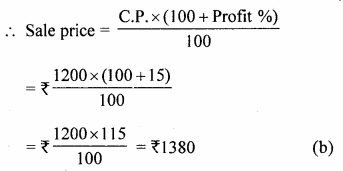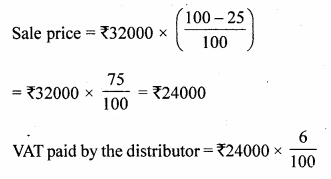# ML Aggarwal Class 10 Solutions for ICSE Maths Chapter 1 Value Added Tax MCQS

## ML Aggarwal Class 10 Solutions for ICSE Maths Chapter 1 Value Added Tax MCQS

These Solutions are part of ML Aggarwal Class 10 Solutions for ICSE Maths. Here we have given ML Aggarwal Class 10 Solutions for ICSE Maths Chapter 1 Value Added Tax MCQS

More Exercises

A retailer purchases a fan for ₹ 1200 from a wholesaler and sells it to a consumer at 15% profit. If the rate of sales tax (under VAT) at every stage is 8%, then choose the correct answer from the given four options for questions 1 to 5 :

Question 1.
The selling price of the fan by the retailer (excluding tax) is
(a) ₹ 1200
(b) ₹ 1380
(c) ₹ 1490.40
(d) ₹ 11296
Solution:
Cost price of a fan = ₹ 1200
Profit = 15%Question 2.
VAT paid by the wholesaler is
(a) ₹ 96
(b) ₹ 14.40
(c) ₹ 110.40
(d) ₹ 180
Solution:
Rate of VAT =8%
.’. VAT paid by wholesaler = ₹ 1200 x $$\\ \frac { 8 }{ 100 }$$
= ₹ 96 (a)

Question 3.
VAT paid by the retailer
(a) ₹ 180
(b) ₹ 110.40
(c) ₹ 96
(d) ₹ 14.40
Solution:
VAT deducted by the retailer = ₹ $$\\ \frac { 1380\times 8 }{ 100 }$$
VAT paid by wholesalers = ₹ 96 .
Net VAT paid by his = ₹ 110.40 – 96.00
= ₹14.40 (d)

Question 4.
VAT collected by the Government on the sale of fan is
(a) ₹14.40
(b) ₹96
(c) ₹110.40
(d) ₹180
Solution:
VAT collected by the govt, on the sale of fan
= ₹ $$\\ \frac { 11040 }{ 100 }$$
= ₹110.40 (c)

Question 5.
The cost of the fan to the consumer inclusive of tax is
(a) ₹1296
(b) ₹1380
(c) ₹1310.40
(d) ₹1490.40
Solution:
Cost of fan to the consumer inclusive tax
= ₹1380 + 110.40
= ₹ 1490.40 (d)

A shopkeeper bought a TVfrom a distributor at a discount of 25% of the listed price of ₹ 32000. The shopkeeper sells the TV to a consumer at the listed price. If the sales tax (under VAT) is 6% at every stage, then choose the correct answer from the given four options for questions 6 to 8 :

Question 6.
VAT paid by the distributor is
(a) ₹1920
(b) ₹1400
(c) ₹480
(d) ₹8000
Solution:
List price of T.V. set = ₹32000
Discount = 25%
Rate of VAT = 6%= ₹1440 (b)

Question 7.
VAT paid by the shopkeeper is
(a) ₹480
(b) ₹1440
(c) ₹1920
(d) ₹8000
Solution:
Total VAT charged by the shopkeeper
= ₹32000 x $$\\ \frac { 6 }{ 100 }$$
= ₹1920
VAT already paid by distributor = ₹ 1440
Net VAT paid by shopkeeper
= ₹1920 – ₹1440
= ₹480 (a)

Question 8.
The cost of the TV to the consumer inclusive of tax is
(a) ₹8000
(b) ₹32000
(c) ₹33920
(d) none of these
Solution:
Cost of T.V. to the consumer inclusive of VAT = ₹32000 + 1920
= ₹33920 (c)

A wholesaler buys a computer from a manufacturer for ₹ 40000. He marks the price of the computer 20% above his cost price and sells it to a retailer at a discount of 10% on the marked price. The retailer sells the computer to a consumer at the marked price. If the rate of sales tax (under VAT) is 10% at every stage, then choose the correct answer from the given four options for questions 9 to 15 :

Question 9.
The marked price of the computer is
(a) ₹40000
(b) ₹48000
(c) ₹50000
(d) none of these
Solution:
C.R of computer for manufacturer = ₹40000
After marking 20% above the C.R, the price
= ₹40000 x $$\\ \frac { 100+20 }{ 100 }$$
= ₹40000 x $$\\ \frac { 120 }{ 100 }$$
= ₹48000 (b)

Question 10.
Cost of the computer to the retailer (excluding tax) is
(a) ₹36000
(b) ₹40000
(c) ₹43200
(d) ₹47520
Solution:
Rate of discount = 10%
.’. Sales price after discount
= ₹48000 x $$\\ \frac { 100-10 }{ 100 }$$
= ₹48000 x $$\\ \frac { 90 }{ 100 }$$
= ₹43200 (c)

Question 11.
Cost of the computer to the retailer inclusive of tax is
(a) ₹47520
(b) 43200
(c) 44000
(d) none of these
Solution:
Rate of sales tax (VAT) = 10%
Sales tax charged = ₹43200 x $$\\ \frac { 10 }{ 100 }$$
= ₹4320
Cost price of T.V. including S.T.
= ₹43200 + ₹4320
= ₹47520 (a)

Question 12.
VAT paid by the manufacturer is
(a) ₹4000
(b) ₹4320
(c) ₹320
(d) none of these
Solution:
VAT paid by the manufacturer
= ₹40000 x $$\\ \frac { 10 }{ 100 }$$
= ₹4000 (a)

Question 13.
VAT paid by the wholesaler is
(a) ₹4000
(b) ₹4320
(c) ₹320
(d) ₹480
Solution:
VAT paid by the wholesaler = ₹43200 x $$\\ \frac { 10 }{ 100 }$$
= ₹4320
Net VAT paid by = ₹4320 – ₹4000
= ₹320 (c)

Question 14.
VAT paid by the retailer is
(a) ₹4000
(b) ₹4320
(c) ₹320
(d) ₹480
Solution:
VAT paid by retailer = 48000 x $$\\ \frac { 10 }{ 100 }$$ = ₹4800
Net VAT to be paid = ₹4800 – 4320
= ₹480 (d)

Question 15.
Consumer’s cost price inclusive of VAT is
(a) ₹47520
(b) ₹48000
(c) ₹52800
(d) ₹44000
Solution:
Sol. Sale price to consumer = ₹4800
VAT paid by the consumer = ₹48000 x $$\\ \frac { 10 }{ 100 }$$
= ₹4800
Consumers cost price inclusive of VAT = ₹48000 + ₹4800
= ₹52800 (c)

Hope given ML Aggarwal Class 10 Solutions for ICSE Maths Chapter 1 Value Added Tax MCQS are helpful to complete your math homework.

If you have any doubts, please comment below. Learn Insta try to provide online math tutoring for you.